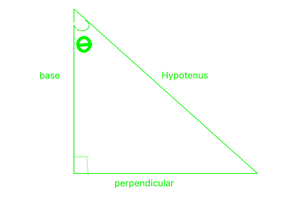# Find the exact value of tan 3π/4.

• Last Updated : 28 Sep, 2021

It is basically a study of the properties of the triangle and trigonometric function and their application in various cases. It helps in finding the angles and missing sides of a triangle with the help of trigonometric ratios. Commonly used angles are  0°, 30°, 45°, 60°, and 90°. With the help of only these angles, find the value of all other trigonometric angles.In this triangle, Given an acute angle θ,

• The sine of θ is written as sinθ and defined as the ratio sinθ = perpendicular/hypotenuse
• The cosine of θ is written as cosθ and defined as the ratio cosθ = base/hypotenuse
• The tangent of θ is written as tanθ and defined as the ratio tanθ = perpendicular/base = sinθ/cosθ

Note The reciprocals of sine, cosine, and tangents also have names: they are cosecant, secant, and cotangent.

• The cosecant of θ is written as cosecθ and defined as cosecθ = 1/sinθ
• The secant of θ is written as secθ and defined as secθ = 1/cosθ
• The cotangent of θ is written as cotθ and defined as cotθ = 1/tanθ

There are three Pythagorean Identities

1. sin2 θ + cos2 θ = 1
2. tan2 θ + 1 = sec2 θ
3. cot2 θ + 1 = cosec2 θ

Lets look at the complementary angles in trigonometric ratios,

• sin(90 + θ) = cosθ
• cos(90 + θ) = -sinθ
• tan(90 + θ) = -cotθ
• cot(90 + θ) = -tanθ
• sec(90 + θ) = -cosecθ
• cosec(90 + θ) = secθ

### Trigonometric Ratio Table

The trigonometric angles have a fixed value. Some of the important angles are used in mathematics. These fixed values are used in calculations. Let’s take a look at the table given below,

### Find the exact value of tan 3π/4.

Solution:

We have to find the value of tan3π/4

tan (3π/4) = tan(π/2 + π/4) = -cot(π/4) [as tan(90 + θ) = -cotθ here θ = π/4]

cot(π/4) = 1

So, tan(3π/4)=-1

Alternate Way

tan(180 – θ) = -tanθ

So, tan(3pi/4) = -tan(pi/4) = -1

### Similar Questions

Question 1: Find the value of tan(5π/6)

Solution:

⇒ tan(5π/6) = tan(π/2 + π/3) = -cot(π/3) [as tan(90 + θ) = -cotθ here θ = π/3]

so cot(π/3) = 1/√3

So, tan(5π/6) = -1/√3

Question 2: Find the value of tan(5π/4)

Solution:

⇒ tan(180 + θ) = tanθ [As tanθ is positive in the third quadrant]

S0, tan(5π/4) = tan(π + π/4) which is equal to tan(π/4)

tan(π/4) = 1

Hence, tan(5π/4) = 1

Question 3: What is the value of cot(5π/6)?

Solution:

⇒ We know that, cotθ = 1/tanθ

tan(5π/6) = -1/√3 [We have deduced earlier]

So, cot(5π/6) = -√3

Alternative way

⇒ cot(5π/6) = cot(π/2 + π/3) = -tan(π/3) [as cot(90 + θ) = -tanθ here θ = π/3]

So tan(π/3) = √3

Hence, cot(5π/6) = -√3

My Personal Notes arrow_drop_up